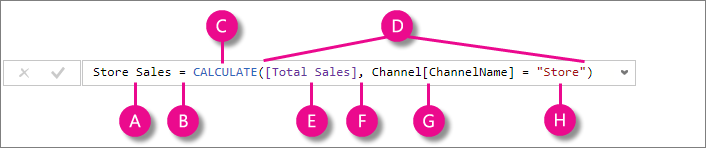# What is DAX:

DAX (Data Analysis Expressions) helps in creating new information from existing information (or) data. The existing information can be at detail level, using DAX we can create information such as aggregated, summarized and so on.

# How to implement DAX:

Before implementing DAX, make sure you know the following details:

• DAX works on column values of a Table.
• DAX cannot modify or insert data, that means source data is SAFE.
• We can create calculated column and measures with DAX but we cannot calculate rows using DAX.

# Sample DAX Formula:This formula includes the following syntax elements:

A. The measure name Total Sales.
B. The equals sign operator (=) indicates the beginning of the formula. When calculated, it will return a result.
C. The DAX function SUM adds up all the numbers in the Sales[SalesAmount] column. You’ll learn more about functions later.
D. Parenthesis () surround an expression containing one or more arguments. All functions require at least one argument. An argument passes a value to a function.
E. The referenced table Sales.
F. The referenced column [SalesAmount] in the Sales table. With this argument, the SUM function knows on which column to aggregate a SUM.

# Most commonly used DAX functions:

• SUM, MIN, MAX, AVG
• COUNTROWS, DISTINCTCOUNT
• IF, AND, OR, SWITCH
• ISBLANK, ISFILTERED, ISCROSSFILTERED
• VALUES, ALL, FILTER, CALCULATE
• UNION, INTERSECT, EXCEPT, NATURALINNERJOIN, SUMMARIZECOLUMNS, NATURALLEFTEROUTERJOIN, ISEMPTY
• MEDIAN, GEOMEAN, DATEDIFF
• VAR (Variables)